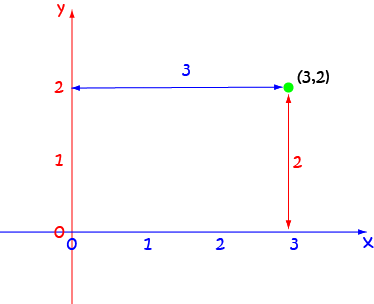# Definition of OrdinateOrdinate is another term for $y$-coordinate.

Every point in the $xy$-plane is represented by an ordered pair, $(x,y)$ that tells us its vertical and horizontal position in the plane.

The ordinate gives the vertical position of the point in the plane. It tells us how far up or down the plane the point lies.

The ordinate always comes second in the ordered pair representing the point.

For example, if the ordered pair $(3,2)$ represents the point $P$ in the $xy$-plane, the ordinate of $P$ is $2$.

### Description

The aim of this dictionary is to provide definitions to common mathematical terms. Students learn a new math skill every week at school, sometimes just before they start a new skill, if they want to look at what a specific term means, this is where this dictionary will become handy and a go-to guide for a student.

### Audience

Year 1 to Year 12 students

### Learning Objectives

Learn common math terms starting with letter O

Author: Subject Coach
You must be logged in as Student to ask a Question.